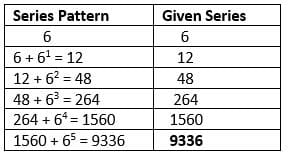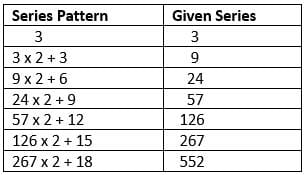Important Questions Test: Number Series- 1

# Important Questions Test: Number Series- 1

Test Description

## 10 Questions MCQ Test Logical Reasoning (LR) and Data Interpretation (DI) | Important Questions Test: Number Series- 1

Important Questions Test: Number Series- 1 for Railways 2023 is part of Logical Reasoning (LR) and Data Interpretation (DI) preparation. The Important Questions Test: Number Series- 1 questions and answers have been prepared according to the Railways exam syllabus.The Important Questions Test: Number Series- 1 MCQs are made for Railways 2023 Exam. Find important definitions, questions, notes, meanings, examples, exercises, MCQs and online tests for Important Questions Test: Number Series- 1 below.
Solutions of Important Questions Test: Number Series- 1 questions in English are available as part of our Logical Reasoning (LR) and Data Interpretation (DI) for Railways & Important Questions Test: Number Series- 1 solutions in Hindi for Logical Reasoning (LR) and Data Interpretation (DI) course. Download more important topics, notes, lectures and mock test series for Railways Exam by signing up for free. Attempt Important Questions Test: Number Series- 1 | 10 questions in 10 minutes | Mock test for Railways preparation | Free important questions MCQ to study Logical Reasoning (LR) and Data Interpretation (DI) for Railways Exam | Download free PDF with solutions
 1 Crore+ students have signed up on EduRev. Have you?
Important Questions Test: Number Series- 1 - Question 1

### Look at this series: 4, 6, 12, 30, 90,  ?, 1260. What number should come?

Detailed Solution for Important Questions Test: Number Series- 1 - Question 1

Logic is:

► 4 × 1.5 = 6,
► 6 × 2 = 12,
► 12 × 2.5 = 30,
► 30 × 3 = 90,
► 90 × 3.5 = 315,
► 315 × 4 = 1260.

Important Questions Test: Number Series- 1 - Question 2

### Look at this series: 7, 10, 8, 11, 9, 12,...so on. What number should come next?

Detailed Solution for Important Questions Test: Number Series- 1 - Question 2

This is a simple alternating addition and subtraction series.

In the first pattern, 3 is added. In the second, 2 is subtracted.

► 7 + 3 = 10,
► 10 - 2 = 8,
► 8 + 3 = 11,
► 11 - 2 = 9,
► 9 + 3 = 12,
► 12 - 2 = 10.

Important Questions Test: Number Series- 1 - Question 3

### Look at this series: 6, 12, 48, 264,1560...so on. What number should come next?

Detailed Solution for Important Questions Test: Number Series- 1 - Question 3Important Questions Test: Number Series- 1 - Question 4

Look at this series: 3, 4, 7, 8, 11, 12,...so on. What number should come next?

Detailed Solution for Important Questions Test: Number Series- 1 - Question 4

This alternating addition series begins with 3.
► Then 1 is added to give 4,
► Then 3 is added to give 7,
► Then 1 is added to give 8,
► Then 3 is added to give 11,
► Then 1 is added to give 12,
► Then 3 is added to give 15.

Important Questions Test: Number Series- 1 - Question 5

Look at this series: 4, 11, 19, 41, ?, 161...so on. What number should come?

Detailed Solution for Important Questions Test: Number Series- 1 - Question 5

Pattern:

► 4 × 2 + 3 = 11,
► 11 × 2 – 3 = 19,
► 19 × 2 + 3 = 41,
► 41 × 2 – 3 = 79,
► 79 × 2 + 3 = 161.

Important Questions Test: Number Series- 1 - Question 6

Look at this series: 53, 53, 40, 40, 27, 27,...so on. What number should come next?

Detailed Solution for Important Questions Test: Number Series- 1 - Question 6

A number is repeated. Then it is reduced by 13 and the result is repeated.

► The next number is 27-13=14.

Important Questions Test: Number Series- 1 - Question 7

Look at this series: 8, 22, 8, 28, 8,...so on. What number should come next?

Detailed Solution for Important Questions Test: Number Series- 1 - Question 7

This is a simple addition series with a random number, 8, interpolated as every other number.

In the series, 6 is added to each number except 8, to arrive at the next number.

► 22 + 6 = 28,
► 28 + 6 = 34.

Important Questions Test: Number Series- 1 - Question 8

Look at this series: 1, 3, 6, 10, 15...so on. What number should come next?

Detailed Solution for Important Questions Test: Number Series- 1 - Question 8

It is a triangular number series forming a triangular pattern.

► 1 = 1,
► 1+2 = 3,
► 1+2+3 = 6,
► 1+2+3+4 = 10,
► 1+2+3+4+5 = 15,
► 1+2+3+4+5+6 = 21.

Important Questions Test: Number Series- 1 - Question 9

Look at this series: 2, 1, (1/2), (1/4),...so on. What number should come next?

Detailed Solution for Important Questions Test: Number Series- 1 - Question 9

This is a simple division series, each number is one-half of the previous number.

In other terms to say, the number is divided by 2 successively to get the next result.

► 4/2 = 2
► 2/2 = 1
► 1/2 = 1/2
► (1/2) / 2 = 1/4
► (1/4) / 2 = 1/8 and so on.

Important Questions Test: Number Series- 1 - Question 10

3, 9, 24, 57, ?, 267, 552. What number should come?

Detailed Solution for Important Questions Test: Number Series- 1 - Question 10## Logical Reasoning (LR) and Data Interpretation (DI)

96 videos|90 docs|119 tests
 Use Code STAYHOME200 and get INR 200 additional OFF Use Coupon Code
Information about Important Questions Test: Number Series- 1 Page
In this test you can find the Exam questions for Important Questions Test: Number Series- 1 solved & explained in the simplest way possible. Besides giving Questions and answers for Important Questions Test: Number Series- 1, EduRev gives you an ample number of Online tests for practice

## Logical Reasoning (LR) and Data Interpretation (DI)

96 videos|90 docs|119 tests